Next:5.1.2 AVL Trees: Insertions and DeletionsUp:5.1 AVL TreesPrevious:5.1 AVL Trees

## 5.1.1 Maximum Height of an AVL Tree

What is the maximum height of an AVL tree having exactly n nodes? To answer this question, we will pose the following question:

What is the minimum number of nodes (sparsest possible AVL tree) an AVL tree of height h can have ?

Let Fh be an AVL tree of height h, having the minimum number of nodes. Fh can be visualized as in Figure 5.2.
Let Fl and Fr be AVL trees which are the left subtree and right subtree, respectively, of Fh. Then Fl or Fr must have height h-2.
Suppose Fl has height h-1 so that Fr has height h-2. Note that Fr has to be an AVL tree having the minimum number of nodes among all AVL trees with height of h-1. Similarly, Fr will have the minimum number of nodes among all AVL trees of height h--2. Thus we have
| Fh| = | Fh - 1| + | Fh - 2| + 1
where | Fr| denotes the number of nodes in Fr. Such trees are called Fibonacci trees. See Figure 5.3. Some Fibonacci trees are shown in Figure 4.20. Note that | F0| = 1 and | F1| = 2.
Adding 1 to both sides, we get
| Fh| + 1 = (| Fh - 1| + 1) + (| Fh - 2| + 1)
Thus the numbers | Fh| + 1 are Fibonacci numbers. Using the approximate formula for Fibonacci numbers, we get
| Fh| + 1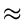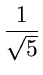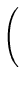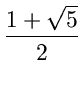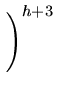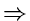h1.44log| Fn|The sparsest possible AVL tree with n nodes has height
h1.44log nThe worst case height of an AVL tree with n nodes is
1.44log n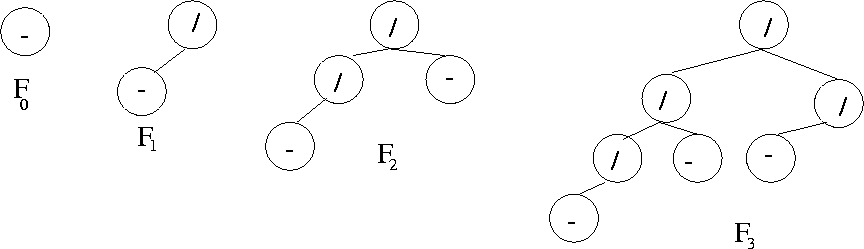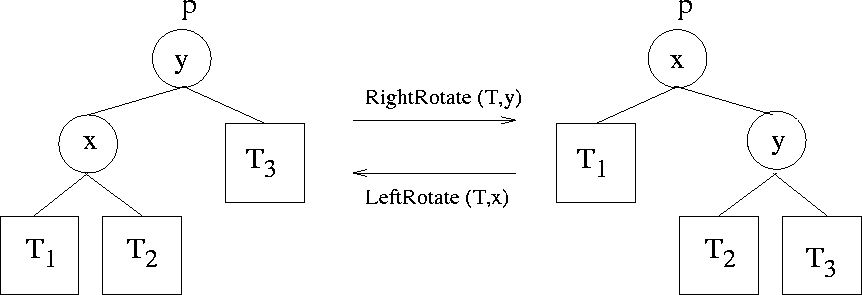Next:5.1.2 AVL Trees: Insertions and DeletionsUp:5.1 AVL TreesPrevious:5.1 AVL Trees
eEL,CSA_Dept,IISc,Bangalore